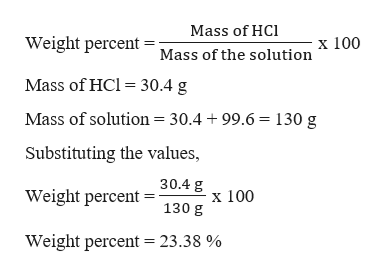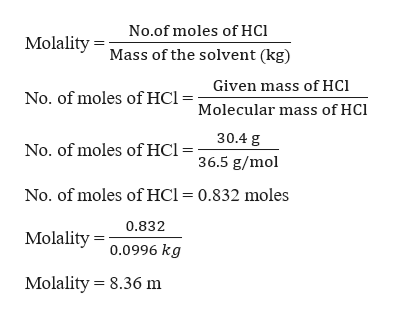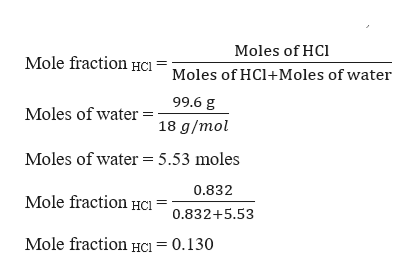# A solution consists of 30.4 g of hydrochloric acid, HCl, and 99.6 g water and has a density of 1.12 g/mL.Calculate the weight percent of HCl, the molality of HCl, the mole fraction of HCl, and the molarity of HCl in the solution.Answer:weight percent =________%molality =______ mmole fraction =_______molarity =____ M

Question
27 views

A solution consists of 30.4 g of hydrochloric acidHCl, and 99.6 g water and has a density of 1.12 g/mL.

Calculate the weight percent of HCl, the molality of HCl, the mole fraction of HCl, and the molarity of HCl in the solution.

 weight percent = ________% molality = ______ m mole fraction = _______ molarity = ____ M
check_circle

Step 1

The weight percent of HCl can be calculated as,help_outlineImage TranscriptioncloseMass of HC Weight percent Mass of the solution x 100 Mass of HCl = 30.4 g Mass of solution = 30.4 +99.6 = 130 g Substituting the values, Weight percent = 30.4 g 130g 100 Weight percent = 23.38 % fullscreen
Step 2

Now let us calculate the molality, which can be calculated as,help_outlineImage TranscriptioncloseNo.of moles of HCI Molality Mass of the solvent (kg) Given mass of HC No. of moles of HCI= Molecular mass of HCl 30.4 g No. of moles ofHCI= 36.5 g/mol No. of moles of HCl = 0.832 moles 0.832 Molality0.0996 kg Molality 8.36 m fullscreen
Step 3

Now let us calculate the mole fraction...help_outlineImage TranscriptioncloseMoles of HCl Mole fraction Hci Moles of HCl+Moles of water 99.6 g Moles of water 18 g/mol 5.53 moles Moles of water 0.832 Mole fraction HC1 0.832+5.53 Mole fraction HCı= 0.130 fullscreen

### Want to see the full answer?

See Solution

#### Want to see this answer and more?

Solutions are written by subject experts who are available 24/7. Questions are typically answered within 1 hour.*

See Solution
*Response times may vary by subject and question.
Tagged in

### Solutions